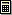Conversion Calculators :: from The Quality PortalMeasurement is as old as history. There are 3 systems of measurement: the English, the Metric and the System International D'unites (or SI). When America separated from England, the colonies retained the English system - at about the same time the metric system was developed in France. This metric system was later modified to develop the SI system. Here we have some conversion calculators for weight, volume, length and pressure.

This is how these work:  just type a number in the field you know, and press the calculate button. It will convert the units for the rest of the boxes. Note that the units are converted first to the first box of each table, and then converted to the respective unit. This double conversion is why if you put in 1 gal, you will not get exactly 8 pints.Weight | Volume | Length | Pressure | Energy | Calorific Volume | Velocity | Temperature calculators available below

Other Calculators:
Easy Unit Converter | Ecoterr Conversion Tools | Monachos' Calculators | Statistics Calculators from UCLA & from CSBSJ |
Standard Deviation Calculator | Calculators from CSG Network | Business Calculators from Peacock Capital | AskNumbers.com
Suggest a site

 Kilograms Ounces Pounds Troy Pounds Stones Short Tons Long Tons
 A pound is a unit of force and a kilogram is a unit of mass. This conversion implies that 1 lb of weight (which is a force) equals the weight exerted by 0.4536 kg of mass assuming the gravity is 9.8m/s2. On the moon, a kilogram will weigh less but still have the same mass.
 Volume Conversion Calculator Liters Fluid Ounces Quarts Gallons Imperial Gallons Pints Table Spoons Tea Spoons
 Length Conversion Calculator Meters Inches Feet Yards Miles Nautical Miles Microns
 Pressure Conversion Calculator Atmospheres In Hg In H2O PSI mm Hg Pascal MPa
 Energy Conversion Calculator Btu Calories/Gram Watt Hour Kilowatt Hour Joule
 Calorific Volume Conversion Calculator Btu/ft3 kcal/m3 W/m3 J/m3
 Velocity Conversion Calculator Meters per Second Miles per Hour Feet per Minute Feet per Second Inches per Second Kilometers per Hour Knots
 Temperature Conversion Calculator [provided by V Moore] Celsius Fahrenheit Kelvin Rankine